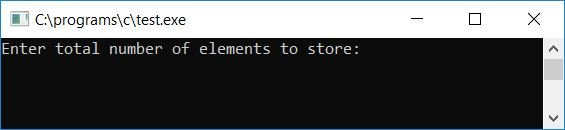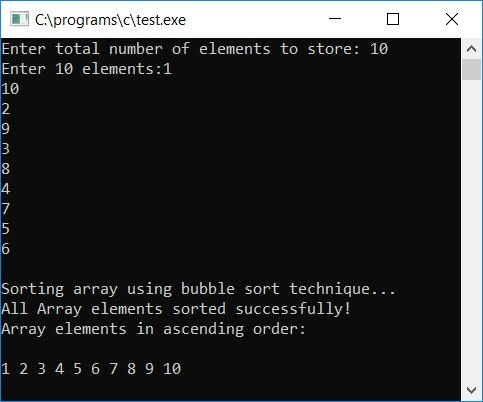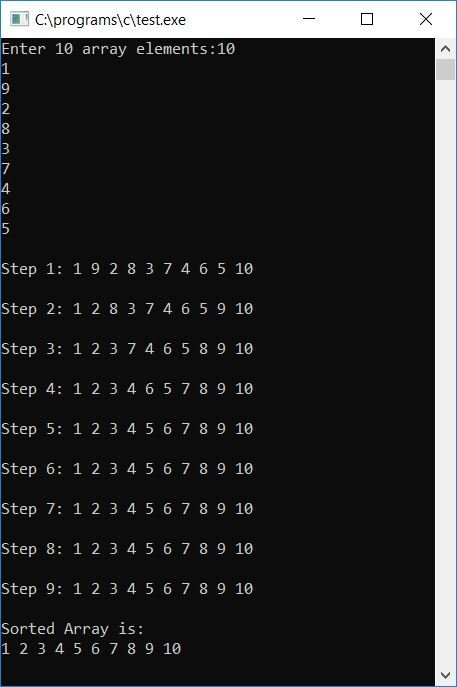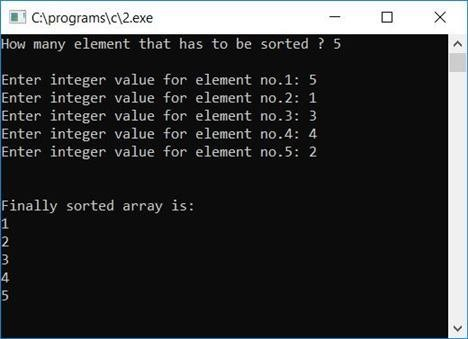# Bubble sort program in C

In this tutorial, we will learn how to create a program in C that sorts an array in ascending order using the bubble sort technique. At last, we have also created a function that can be used to sort any given array in ascending order.

However, before proceeding with the program, if you are unfamiliar with how bubble sorting works, please refer to the step-by-step operation of bubble sort. Now let's move on and implement it in the C program.

## Bubble Sort in C

Now let's implement bubble sort in a C program as given below. I'll explain each and every line of code later on. The question is: write a program in C that sorts a given array in ascending order using the bubble sort technique. The answer to this question is:

```#include<stdio.h>
#include<conio.h>
int main()
{
int arr, i, j, n, temp;
printf("Enter total number of elements to store: ");
scanf("%d", &n);
printf("Enter %d elements:", n);
for(i=0; i<n; i++)
scanf("%d", &arr[i]);
printf("\nSorting array using bubble sort technique...\n");
for(i=0; i<(n-1); i++)
{
for(j=0; j<(n-i-1); j++)
{
if(arr[j]>arr[j+1])
{
temp = arr[j];
arr[j] = arr[j+1];
arr[j+1] = temp;
}
}
}
printf("All Array elements sorted successfully!\n");
printf("Array elements in ascending order:\n\n");
for(i=0; i<n; i++)
printf("%d ", arr[i]);
getch();
return 0;
}```

As the above program was written in the Code::Blocks IDE, here is the first snapshot of the sample run:Now supply the size of the array, say 10, and then enter 10 array elements. After providing the array size and its element, press the ENTER key to sort and print all array elements in ascending order using the bubble sort method. Here is the second snapshot of the sample run:#### Program Explained

• We asked the user to enter the size of the array.
• Then, enter array elements of that size.
• Now for sorting, we have used first (outer) for the loop from 0 to (n-1) as array indexing starts at 0, not 1.
• Then comes the second (inner) for loop from 0 to (n-i-1). As we have to compare two consecutive elements, if the first element is greater than the second, then we have to swap.
• For example, if the value of i is 0, then the value of j will also be 0, and then using an if statement, the 0th element is compared with the (0+1)th or 1st element. If the element present at 0 is greater than the element present at 1, then the swap will be performed.
• If there are a total of 10 elements in the array, the loop will run until the 8th element is compared with the (8+1)th element, that is, the 9th element, which is the last one.
• After running the loop to check, compare, and swap every consecutive element (or every jth to (j+1)th element), we have sorted the array in ascending order.
• Finally, print out the same array, which will show all of its elements in ascending order.

### C bubble sort: show array after each sort

If you want to display the array after each sort, then here is the program you may go for:

```#include<stdio.h>
#include<conio.h>
int main()
{
int arr, i, j, temp;
printf("Enter 10 array elements:");
for(i=0; i<10; i++)
scanf("%d", &arr[i]);
for(i=0; i<(10-1); i++)
{
for(j=0; j<(10-i-1); j++)
{
if(arr[j]>arr[j+1])
{
temp = arr[j];
arr[j] = arr[j+1];
arr[j+1] = temp;
}
}
printf("\n");
printf("Step %d: ", i+1);
for(j=0; j<10; j++)
printf("%d ", arr[j]);
printf("\n");
}
printf("\nSorted Array is:\n");
for(i=0; i<10; i++)
printf("%d ", arr[i]);
getch();
return 0;
}```

Here is the final snapshot of this program:### Function-based Bubble Sort Program in C

Here is another program that does the same job using a user-defined function, bubbleSort():

```#include<stdio.h>
#include<conio.h>
void bubbleSort(int arr[], int n);
int main()
{
int arr, nos, i;
printf("How many element that has to be sorted ? ");
scanf("%d", &nos);
printf("\n");
for(i=0; i<nos; i++)
{
printf("Enter integer value for element no.%d: ",i+1);
scanf("%d",&arr[i]);
}
bubbleSort(arr, nos);
printf("\n\nFinally sorted array is:\n");
for(i=0; i<nos; i++)
printf("%d\n",arr[i]);
getch();
return 0;
}
void bubbleSort(int arr[], int no)
{
int i, j, temp;
for(i=no-2; i>=0; i--)
{
for(j=0; j<=i; j++)
{
if(arr[j]>arr[j+1])
{
temp = arr[j];
arr[j] = arr[j+1];
arr[j+1] = temp;
}
}
}
}```

After a successful build and run, Here is the first screenshot of the sample run:And here is the second screenshot of the sample run:#### Program Explained

Except for the function, all of the steps are the same as those discussed in the first program (of this article). As here, we have used functions to implement bubble sort. Here are some of the main steps for using function in this case:

• Here we have asked the user to enter an array size and then all elements of that size one by one using a for loop.
• The array and array size have been passed to function.Passing array means passing array elements; array sizes enclosed in square brackets, such as arr, are optional.
• Following the first two steps, we arrive at the function step. In terms of function, we have used the same technique as discussed above, which is comparing each consecutive element from start to finish.
• If first is greater than second, then swap; if second is greater than third, then swap; and so on.
• But here we have initialized i with (no-1). The word "no" denotes the total number of elements. For example, if the total number of elements is 10, then i is initialized to 8, and in the second loop, j is initialized with 0.
• Then, at first run, the jth element is compared with the (j+1)th element. In other words, the 0th element is compared to the 1st element. The first element is compared to the second element in the second run, and so on. This loop will run a total of eight times to compare the 8th element with the 9th element, which is the last element.
• After successfully completing all the above steps, the function execution will be over, and our array will be sorted in ascending order.
• Now we only have to display the array element with the help of the loop.

#### The same program in different languages

C Quiz

« Previous Program Next Program »